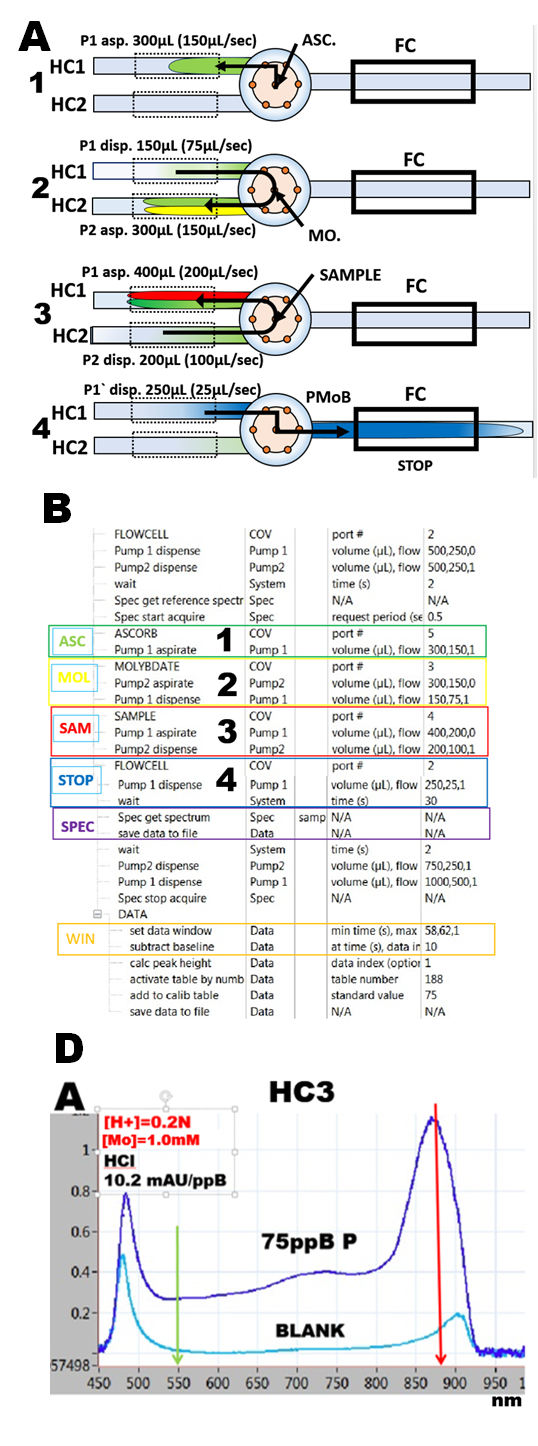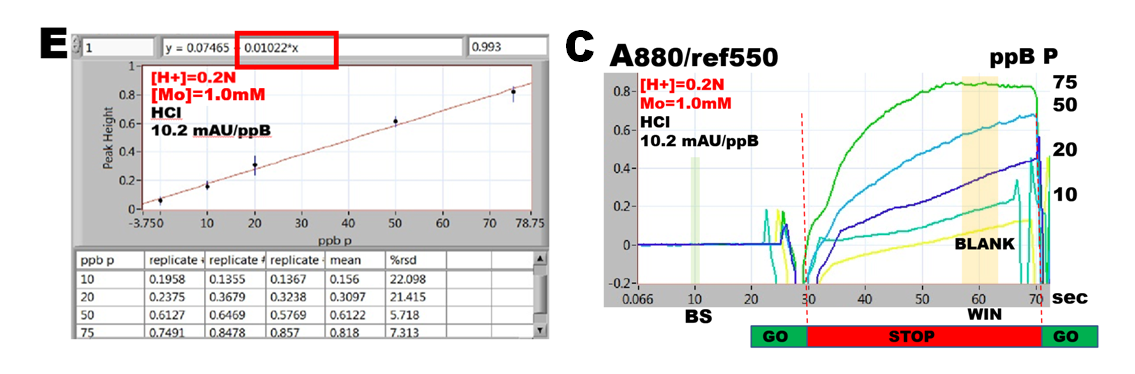Flow Programming and Data Collection

In order to identify conditions at which the molar absorptivity of PMoB is maximized, the flow programming must be designed to reproduce conditions equal to batch measurement, where reactants of well defined concentrations are homogenously mixed. Therefore the microfluidic manipulations (A) and the assay protocol (B) are devised here in the way, explained in (1.5.2.) where, in sections (1.5.3. and 1.5.4.) this approach was used for determination of molar absorptivity.

Thus, for PMoB method, ascorbic acid (step 1), is mixed with molybdate reagent in proportion 1+1 (step 2), followed by step 3, where 200 mcrL sample is mixed with 200 mcrL of the ascorbic acid/molybdate reagent. Next (step 4) the reactant mixture is transported into flow cell for 30 sec long incubation time, during which reaction rates are recorded (C) and, at the end of which, the absorbance is being measured (WIN) along with VIS spectrum (D) of the reactants. Repetition of this protocol with phosphate standards in the range 0 to 75 ppB P yields a calibration line (E), the slope of which is used to compute molar absorptivity.

Each point on the  [H+] diagrams presented on the next three pages represents the slope of calibration line obtained in this way, and is also associated with corresponding spectra and reaction rate curves.

1.5.9.